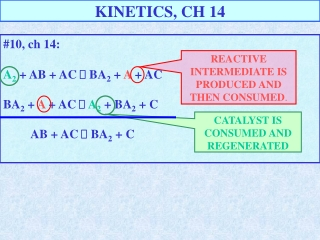DownloadDownload PresentationKINETICS, CH 14

# KINETICS, CH 14

Download Presentation## KINETICS, CH 14

- - - - - - - - - - - - - - - - - - - - - - - - - - - E N D - - - - - - - - - - - - - - - - - - - - - - - - - - -
##### Presentation Transcript

1. KINETICS, CH 14 #10, ch 14: A2 + AB + AC  BA2 + A + AC BA2 + A + AC  A2 + BA2 + C AB + AC  BA2 + C REACTIVE INTERMEDIATE IS PRODUCED AND THEN CONSUMED. CATALYST IS CONSUMED AND REGENERATED

2. #16 SLOPE OF LINES GIVES RATES: AT 75 MINRATE IS 4.2 * 10-5 M/min. OR 7.0 * 10-5 M/sec AT 250 MIN RATE IS 2.1 * 10-3 M/min (3.5 * 10-3 M/sec )

3. #20, ch 14: 2 THE C2H4 DISSAPEARS AT A RATE OF 0.37 M/s, AND WATER AND CO2 APPEAR TWICE AS FAST 2(0.37 M/s) = 0.74 M/s. [CO2]/2 t [H2O]/ t - [C2H4]/ t - [C2H4]/ t [H2O]/2 t = = [CO2]/ t = = INDICATES C2H4 NEEDS TWICE THE TIME TO DISSAPEAR AS IT TAKES FOR CO2 AND H2O TO APPEAR. INDICATES THE CO2 AND H2O APPEAR IN ½ THE TIME (TWICE AS FAST) AS IT TAKES THEC2H4 TO DISSAPEAR. C2H4 +3O2 2CO2 + 2 H2O N2H4 +H2 2NH3 N2H4 IS CONSUMED AT AT RATE OF 63 TORR/hr, THUS NH3 IS PRODUCES AT DOUBLE THAT RATE: 2(63 TORR/hr) = 126 TORR/hr

4. #32 CH 14 S2O82-+3 I- 2 SO42-+ I3- [S2O82-] INCRESES A FACTOR OF 1.5 WHILE THE RATE INCRESES BY 1.5: S2O82- IS FIRST ORDER.

5. #32 CH 14 S2O82-+3 I- 2 SO42-+ I3- [S2O82-] INCRESES A FACTOR OF 2, I- BY A FACTOR OF1.5, WHILE THE RATE INCRESES BY 3 FOLD: S2O82- IS FIRST ORDER(KNOWN) AND I- MUST BE FIRST ORDER. 2 * 1.5 = 3 : 31 = 3 The exponent of 1 makes the equation true, the reactants are first order

6. #32-B S2O82-+3 I- 2 SO42-+ I3- RATE=k [S2O82-][I-] ,VALUES FROM TABLE SUBSTITUTED IN. AVERAGE k = 4.0 * 10-3 M-1 s-1 #32-C DISSAPEARS 1/3 AS RAPIDLY AS I- , THEREFORE RATE = 3 (4.0 * 10-3)(0.015 M )(0.040 M) = 7.2 * 10-6 M/s = RATE OF I- DISSAPEARANCE S2O82-

7. #42 CH 14 Plot of ln A vs t is linear,Reaction is 1st order in A 1/mol(A) ln(A) t

8. #42 CH 14-b 42-b k = -slope k = -[-3.91 –(-2.70)]/120 = 0.0101 s-1 Plot of ln A vs t is linear,Reaction is 1st order in A ln A 42-c t1/2 = 0.693/k = 0.693/0.0101 = 68.7s ln A

9. #48-a CH 14 f =e-Ea/RT a) -Ea/RT = 1.60 J/mol x mol*K = -38.489 500K8.314 J f =e-38.489 = 1.92 * 10-17 b) -Ea/RT = 1.60 J/mol x mol*K = -37.735 510K8.314 J f =e-37.735 = 4.09 * 10-17 4.09 * 10-17 / 1.92 * 10-17 = 2.13 times more at 510K

10. #54 CH 14-b ln [k1/k2]= Ea/R [1/T2 – 1/T1] ln [0.0796/0.0815]= Ea/8.314J/mol [1/1220 – 1/1010] -0.023589 = Ea (-1.704 * 10-4) / 8.313 J/mol Ea = 8.313(-0.023589)J/mol = 1.151 * 103 J/mol

11. #68 CH 14 • HBr + O2 HOOBr (SLOW) • HOOBr + HBr  2 HOBr • 2 HOBr + 2 HBr  2 H2O + 2 Br2 • 4 HBr + O2 2H2O + Br2 b) GIVEN VERBALLY IN PROBLEM RATE = [HBr][O2], BOTH FIRST ORDER. THIS AGGREES WITH STEP 1) WHICH MUST BE SLOW. c) HOOBr AND HOBr ARE INTERMEDIATES d) THE INTERMEDIATES MY NOT ACCUMULATE IF THEY ARE IN A FAST STEP AFTER A SLOW ONE AS HERE.

12. #72 CH 14 • 2 [ N2O + NO  N2 + NO2] • 2 NO2  2 NO + O2 • 2 N2O  2N2 + O2 INTERMEDIATE CATALYST • 2 N2O + 2 NO 2 N2 + 2 NO2 (SLOW) • 2 NO2  2 NO + O2 • 2 N2O  2N2 + O2 THE INTERMEDIATE NO2 DOES NOT ACCUMULATE AS IT IS PRODUCED IN THE SKOW STEP AND CONSUMED IN THE FAST STEP

13. #80 CH 14 ln kc – ln k = [ Ea – Eac/RT] or Ea-Eac = RT ln (kc/k) Where c is with catalyst. Represents the ratio of rates asked for in problem. Ea-Eac = RT ln (kc/k) Ea-Eac =8.314 J * 310K * ln (1 * 105) Ea-Eac =2.966 * 104 = 30. kJ